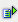The function sum (range) calculates the sum of the values within a range.

Syntax: =sum(range)Example

Cells A1 to A10: any numbers

Cell B1 = sum(A1:A10)

 R / C A B 1 1 55 2 2 3 3 4 4 5 5 6 6 7 7 8 8 9 9 10 10